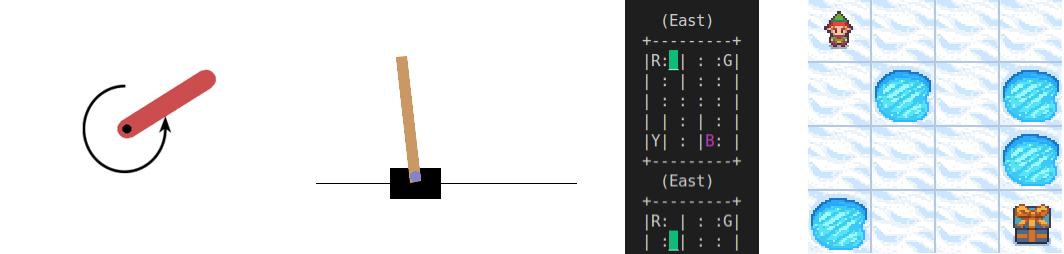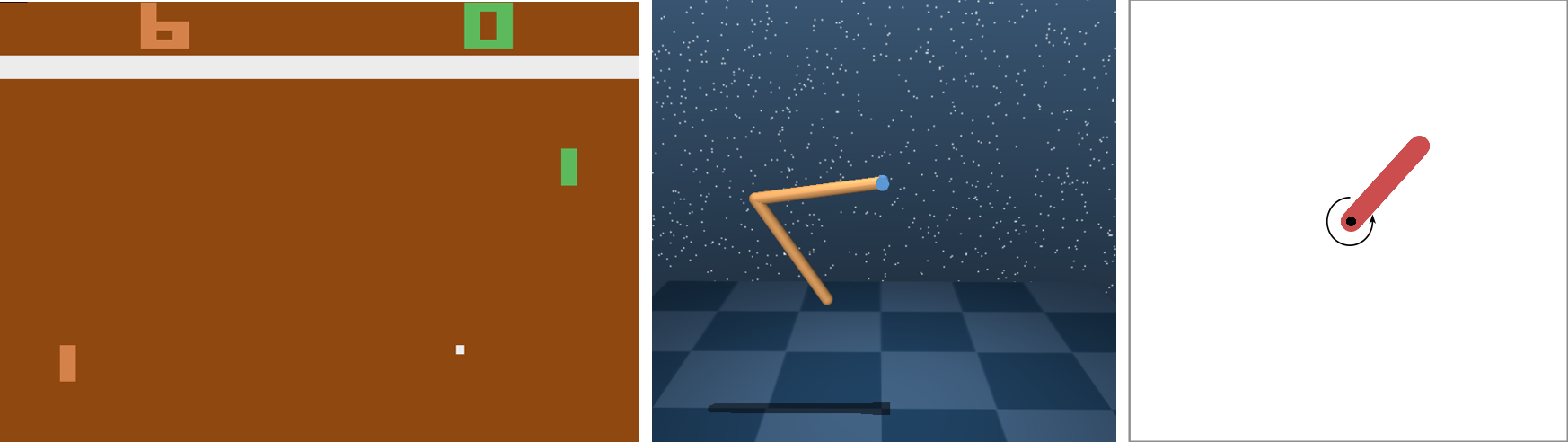# Examples#

In this section, you will find a variety of examples that demonstrate how to use this library to solve reinforcement learning tasks. With the knowledge and skills you gain from trying these examples, you will be well on your way to using this library to solve your reinforcement learning problems.

Note

## Gymnasium / Gym#

### Gymnasium / Gym environments#

Training/evaluation of an agent in Gymnasium / Gym environments (one agent, one environment)Benchmark results are listed in Benchmark results #32 (Gymnasium/Gym)

Note

(*) The examples use a wrapper around the original environment to mask the velocity in the observation. The intention is to make the MDP partially observable and to show the capabilities of recurrent neural networks

### Gymnasium / Gym vectorized environments#

Training/evaluation of an agent in Gymnasium / Gym vectorized environments (one agent, multiple independent copies of the same environment in parallel)

### Shimmy (API conversion)#

The following examples show the training in several popular environments (Atari, DeepMind Control and OpenAI Gym) that have been converted to the Gymnasium API using the Shimmy (API conversion tool) packageNote

From skrl, no extra implementation is necessary, since it fully supports Gymnasium API

Note

Because the Gymnasium API requires that the rendering mode be specified during the initialization of the environment, it is not enough to set the headless option in the trainer configuration to render the environment. In this case, it is necessary to call the gymnasium.make function using render_mode="human" or any other supported option

## Other supported APIs#

### DeepMind environments#

These examples perform the training of one agent in a DeepMind environment (one agent, one environment)Environment

Script

Checkpoint (Hugging Face)

Control: Cartpole SwingUp

dm_suite_cartpole_swingup_ddpg.py

Manipulation: Reach Site Vision

dm_manipulation_stack_sac.py

### Robosuite environments#

These examples perform the training of one agent in a robosuite environment (one agent, one environment)Environment

Script

Checkpoint (Hugging Face)

TwoArmLift

td3_robosuite_two_arm_lift.py

### Bi-DexHands environments#

Multi-agent training/evaluation in a Bi-DexHands environmentEnvironment

Script

Checkpoint (Hugging Face)

## NVIDIA Isaac Gym preview#

### Isaac Gym environments#

Training/evaluation of an agent in Isaac Gym environments (one agent, multiple environments)The agent configuration is mapped, as far as possible, from the IsaacGymEnvs configuration for rl_games. Shared models or separated models are used depending on the value of the network.separate variable. The following list shows the mapping between the two configurations:

# memory
memory_size = horizon_length

# agent
rollouts = horizon_length
learning_epochs = mini_epochs
mini_batches = horizon_length * num_actors / minibatch_size
discount_factor = gamma
lambda = tau
learning_rate = learning_rate
learning_rate_scheduler_kwargs = {"kl_threshold": kl_threshold}
random_timesteps = 0
learning_starts = 0
ratio_clip = e_clip
value_clip = e_clip
clip_predicted_values = clip_value
entropy_loss_scale = entropy_coef
value_loss_scale = 0.5 * critic_coef
kl_threshold = 0
rewards_shaper = lambda rewards, timestep, timesteps: rewards * scale_value

# trainer
timesteps = horizon_length * max_epochs


Benchmark results are listed in Benchmark results #32 (NVIDIA Isaac Gym)

Note

Isaac Gym environments implement a functionality to get their configuration from the command line. Because of this feature, setting the headless option from the trainer configuration will not work. In this case, it is necessary to invoke the scripts as follows: python script.py headless=True for Isaac Gym environments (preview 3 and preview 4) or python script.py --headless for Isaac Gym environments (preview 2)

## NVIDIA Isaac Orbit#

### Isaac Orbit environments#

Training/evaluation of an agent in Isaac Orbit environments (one agent, multiple environments)The agent configuration is mapped, as far as possible, from the Isaac Orbit configuration for rl_games. Shared models or separated models are used depending on the value of the network.separate variable. The following list shows the mapping between the two configurations:

# memory
memory_size = horizon_length

# agent
rollouts = horizon_length
learning_epochs = mini_epochs
mini_batches = horizon_length * num_actors / minibatch_size
discount_factor = gamma
lambda = tau
learning_rate = learning_rate
learning_rate_scheduler_kwargs = {"kl_threshold": kl_threshold}
random_timesteps = 0
learning_starts = 0
ratio_clip = e_clip
value_clip = e_clip
clip_predicted_values = clip_value
entropy_loss_scale = entropy_coef
value_loss_scale = 0.5 * critic_coef
kl_threshold = 0
rewards_shaper = lambda rewards, timestep, timesteps: rewards * scale_value

# trainer
timesteps = horizon_length * max_epochs


Benchmark results are listed in Benchmark results #32 (NVIDIA Isaac Orbit)

Note

Isaac Orbit environments implement a functionality to get their configuration from the command line. Because of this feature, setting the headless option from the trainer configuration will not work. In this case, it is necessary to invoke the scripts as follows: orbit -p script.py --headless

## NVIDIA Omniverse Isaac Gym#

### Omniverse Isaac Gym environments (OIGE)#

Training/evaluation of an agent in Omniverse Isaac Gym environments (OIGE) (one agent, multiple environments)The agent configuration is mapped, as far as possible, from the OmniIsaacGymEnvs configuration for rl_games. Shared models or separated models are used depending on the value of the network.separate variable. The following list shows the mapping between the two configurations:

# memory
memory_size = horizon_length

# agent
rollouts = horizon_length
learning_epochs = mini_epochs
mini_batches = horizon_length * num_actors / minibatch_size
discount_factor = gamma
lambda = tau
learning_rate = learning_rate
learning_rate_scheduler_kwargs = {"kl_threshold": kl_threshold}
random_timesteps = 0
learning_starts = 0
ratio_clip = e_clip
value_clip = e_clip
clip_predicted_values = clip_value
entropy_loss_scale = entropy_coef
value_loss_scale = 0.5 * critic_coef
kl_threshold = 0
rewards_shaper = lambda rewards, timestep, timesteps: rewards * scale_value

# trainer
timesteps = horizon_length * max_epochs


Benchmark results are listed in Benchmark results #32 (NVIDIA Omniverse Isaac Gym)

Note

Omniverse Isaac Gym environments implement a functionality to get their configuration from the command line. Because of this feature, setting the headless option from the trainer configuration will not work. In this case, it is necessary to invoke the scripts as follows: python script.py headless=True

### Omniverse Isaac Gym environments (simultaneous learning by scope)#

Simultaneous training/evaluation by scopes (subsets of environments among all available environments) of several agents in the same run in OIGE’s Ant environment (multiple agents and environments)Three cases are presented:

• Simultaneous (sequential) training of agents that share the same memory and whose scopes are automatically selected to be as equal as possible.

• Simultaneous (sequential) training of agents without sharing memory and whose scopes are specified manually.

• Simultaneous (parallel) training of agents without sharing memory and whose scopes are specified manually.

Note

Omniverse Isaac Gym environments implement a functionality to get their configuration from the command line. Because of this feature, setting the headless option from the trainer configuration will not work. In this case, it is necessary to invoke the scripts as follows: python script.py headless=True

### Omniverse Isaac Sim (single environment)#

Training/evaluation of an agent in Omniverse Isaac Sim environment implemented using the Gym interface (one agent, one environment)

This example performs the training of an agent in the Isaac Sim’s Cartpole environment described in the Creating New RL Environment tutorial

Use the steps described below to setup and launch the experiment after follow the tutorial

# download the sample code from GitHub in the directory containing the cartpole_task.py script
wget https://raw.githubusercontent.com/Toni-SM/skrl/main/docs/source/examples/isaacsim/torch_isaacsim_cartpole_ppo.py

# run the experiment
PYTHON_PATH torch_isaacsim_cartpole_ppo.py


Environment

Script

Checkpoint (Hugging Face)

Cartpole

torch_isaacsim_cartpole_ppo.py

## Real-world examples#

These examples show basic real-world and sim2real use cases to guide and support advanced RL implementations

3D reaching task (Franka’s gripper must reach a certain target point in space). The training was done in Omniverse Isaac Gym. The real robot control is performed through the Python API of a modified version of frankx (see frankx’s pull request #44), a high-level motion library around libfranka. Training and evaluation is performed for both Cartesian and joint control space

Implementation (see details in the table below):

• The observation space is composed of the episode’s normalized progress, the robot joints’ normalized positions ($$q$$) in the interval -1 to 1, the robot joints’ velocities ($$\dot{q}$$) affected by a random uniform scale for generalization, and the target’s position in space ($$target_{_{XYZ}}$$) with respect to the robot’s base

• The action space, bounded in the range -1 to 1, consists of the following. For the joint control it’s robot joints’ position scaled change. For the Cartesian control it’s the end-effector’s position ($$ee_{_{XYZ}}$$) scaled change. The end-effector position frame corresponds to the point where the left finger connects to the gripper base in simulation, whereas in the real world it corresponds to the end of the fingers. The gripper fingers remain closed all the time in both cases

• The instantaneous reward is the negative value of the Euclidean distance ($$\text{d}$$) between the robot end-effector and the target point position. The episode terminates when this distance is less than 0.035 meters in simulation (0.075 meters in real-world) or when the defined maximum timestep is reached

• The target position lies within a rectangular cuboid of dimensions 0.5 x 0.5 x 0.2 meters centered at (0.5, 0.0, 0.2) meters with respect to the robot’s base. The robot joints’ positions are drawn from an initial configuration [0º, -45º, 0º, -135º, 0º, 90º, 45º] modified with uniform random values between -7º and 7º approximately

Variable

Formula / value

Size

Observation space

$$\dfrac{t}{t_{max}},\; 2 \dfrac{q - q_{min}}{q_{max} - q_{min}} - 1,\; 0.1\,\dot{q}\,U(0.5,1.5),\; target_{_{XYZ}}$$

18

Action space (joint)

$$\dfrac{2.5}{120} \, \Delta q$$

7

Action space (Cartesian)

$$\dfrac{1}{100} \, \Delta ee_{_{XYZ}}$$

3

Reward

$$-\text{d}(ee_{_{XYZ}},\; target_{_{XYZ}})$$

Episode termination

$$\text{d}(ee_{_{XYZ}},\; target_{_{XYZ}}) \le 0.035 \quad$$ or $$\quad t \ge t_{max} - 1$$

Maximum timesteps ($$t_{max}$$)

100

Workflows:

Warning

Make sure you have the e-stop on hand in case something goes wrong in the run. Control via RL can be dangerous and unsafe for both the operator and the robot

Target position entered via the command prompt or generated randomly

Target position in X and Y obtained with a USB-camera (position in Z fixed at 0.2 m)

Prerequisites:

A physical Franka Emika Panda robot with Franka Control Interface (FCI) is required. Additionally, the frankx library must be available in the python environment (see frankx’s pull request #44 for the RL-compatible version installation)

Files

Evaluation:

python3 reaching_franka_real_skrl_eval.py


Main environment configuration:

Note

In the joint control space the final control of the robot is performed through the Cartesian pose (forward kinematics from specified values for the joints)

The control space (Cartesian or joint), the robot motion type (waypoint or impedance) and the target position acquisition (command prompt / automatically generated or USB-camera) can be specified in the environment class constructor (from reaching_franka_real_skrl_eval.py) as follow:

control_space = "joint"   # joint or cartesian
motion_type = "waypoint"  # waypoint or impedance
camera_tracking = False   # True for USB-camera tracking


## Library utilities (skrl.utils module)#

This example shows how to use the library utilities to carry out the post-processing of files and data generated by the experimentsExample of a figure, generated by the code, showing the total reward (left) and the mean and standard deviation (right) of all experiments located in the runs folder

tensorboard_file_iterator.py

Note: The code will load all the Tensorboard files of the experiments located in the runs folder. It is necessary to adjust the iterator’s parameters for other paths

import numpy as np
import matplotlib.pyplot as plt

from skrl.utils import postprocessing

labels = []
rewards = []

# load the Tensorboard files and iterate over them (tag: "Reward / Total reward (mean)")
tensorboard_iterator = postprocessing.TensorboardFileIterator("runs/*/events.out.tfevents.*",
tags=["Reward / Total reward (mean)"])
for dirname, data in tensorboard_iterator:
rewards.append(data["Reward / Total reward (mean)"])
labels.append(dirname)

# convert to numpy arrays and compute mean and std
rewards = np.array(rewards)
mean = np.mean(rewards[:,:,1], axis=0)
std = np.std(rewards[:,:,1], axis=0)

# creae two subplots (one for each reward and one for the mean)
fig, ax = plt.subplots(1, 2, figsize=(15, 5))

# plot the rewards for each experiment
for reward, label in zip(rewards, labels):
ax.plot(reward[:,0], reward[:,1], label=label)

ax.set_title("Total reward (for each experiment)")
ax.set_xlabel("Timesteps")
ax.set_ylabel("Reward")
ax.grid(True)
ax.legend()

# plot the mean and std (across experiments)
ax.fill_between(rewards[0,:,0], mean - std, mean + std, alpha=0.5, label="std")
ax.plot(rewards[0,:,0], mean, label="mean")

ax.set_title("Total reward (mean and std of all experiments)")
ax.set_xlabel("Timesteps")
ax.set_ylabel("Reward")
ax.grid(True)
ax.legend()

# show and save the figure
plt.show()
plt.savefig("total_reward.png")